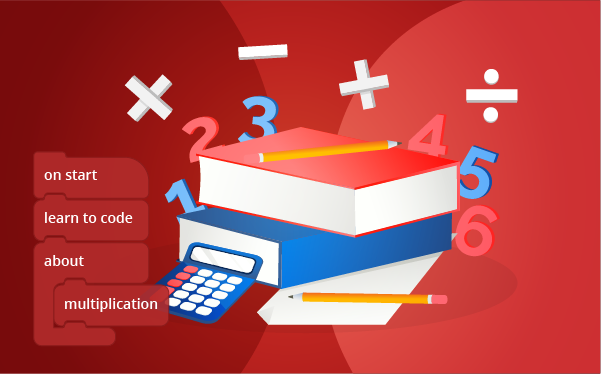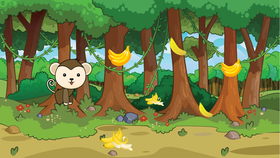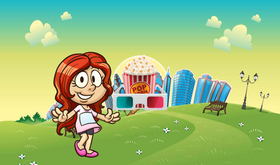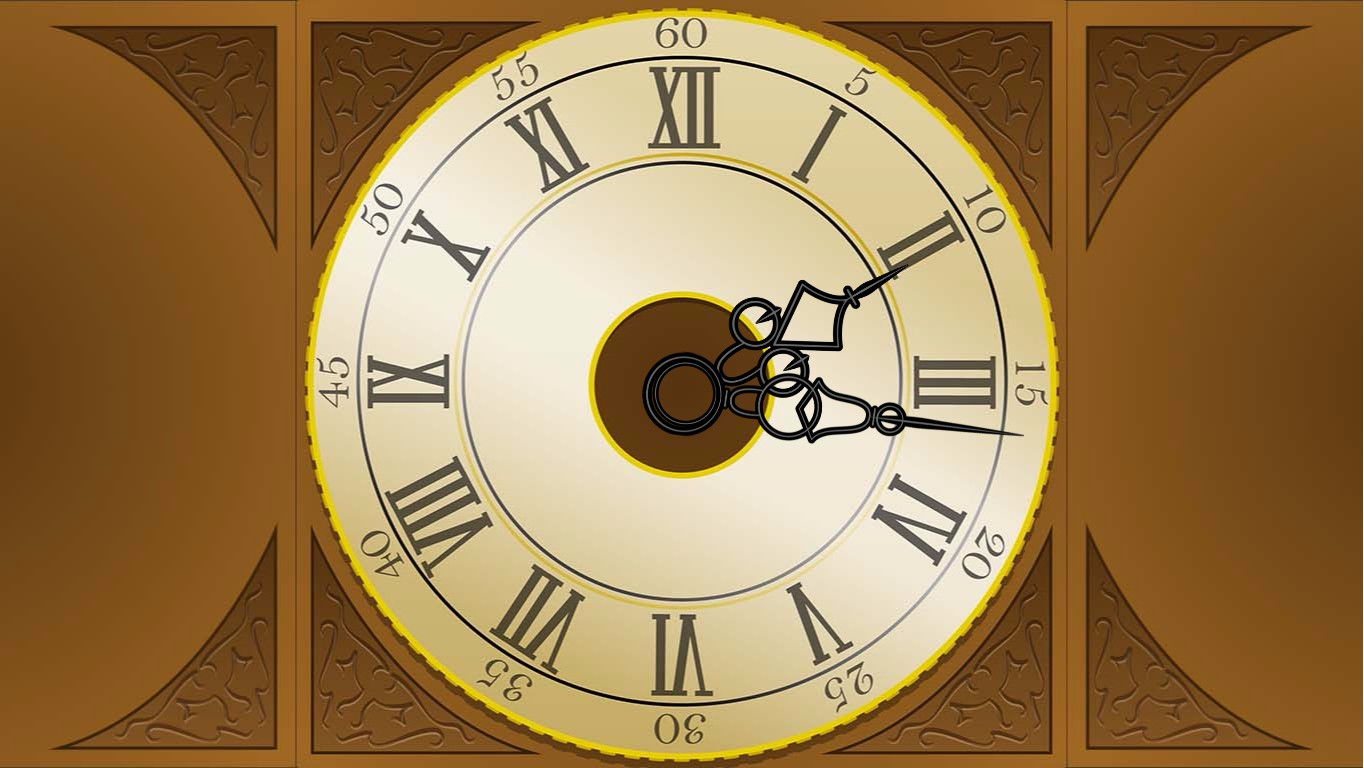« K-8 Courses
This course is included with our Coding/STEAM Curriculum - K-8 Plan# Math 101 Icon Coding 10-Course Pack Space Cadet Dragon Spells Programming 1A Programming 1B Programming 100 Programming 300 Barbie™ You Can Be Anything™ Programming 101 Programming 102 Programming 201 Programming 202 Programming 301 Programming 302 JavaScript 101 Python 101 Web Development 101 Python 201 Drones 101 WeDo Coding Augmented Reality micro:bit 101 MicroPython 101 Life Science 101 Physical Science 101 Earth Science 101 Math 101 Social Studies 101 English 101 Life Science 201 Physical Science 201 Earth Science 201 Math 201 Social Studies 201 English 201

A collection of elementary math projects that demonstrate the application of coding to illustrate or solve basic arithmetic problems and concepts.

• Beginner

## Description

Once your students have completed at least five lessons of Programming 101 or equivalent coding experience, you can assign these CCSS-aligned projects to complement your teaching on topics in arithmetic, geometry, measurement units, fractions, and more. For example, if you’re teaching a lesson on multiplication, you can assign the Multiplication Escape project. Your students will use coding to create a fun game where the player has to quickly answer multiplication questions to move forward and escape falling rocks. Students can also create projects to model word problems, like making change or dividing candies between a group of friends.

With this collection of math projects, you can easily integrate coding and project-based learning into your curriculum. Each STEM lesson walks students through how to make a project about something they’re learning in school with step-by-step instructions. At each step, it encourages them to make their project unique and interesting, emphasizing that coding is a creative medium much like writing or drawing.

We’re constantly updating our STEM courses with new projects, so if there’s something you’d like us to add, send us a message at support@tynker.com.## What Students Learn

• Use programming for math projects
• Build a slide show on a topic
• Build a quiz game
• Make an interactive chart
• Use animation to illustrate
• Narrate using your own voice
• Use the physics engine to model
• Troubleshoot and debug programs

## Technical Requirements

* Online courses require a modern desktop computer, laptop computer, Chromebook, or Netbook with Internet access and a Chrome (29+), Firefox (30+), Safari (7+), or Edge (20+) browser. No downloads required.
* Tablet courses require an iPad (iOS 10+) with Tynker or Tynker Junior app installed and Internet access

## Lesson Summary

Create a project that demonstrates your knowledge of multiplication.
##### Introduction
In this lesson, students will play a Multiplication Escape game and solve multiplication problems to move the goblin Actor across the Stage. Next, students will follow a step-by-step tutorial in the DIY (do-it-yourself) module to create their own version of the Multiplication Escape game they just played. They will program the logic for the questions, create their own math questions, and code the goblin Actor to move when the answers are correct. If students want to make the game experience more challenging, encourage them to increase the speed of the falling bolder Actors. Or if students want to ask harder math questions, tell them to include division, subtraction, and fraction problems.

After completing the DIY module, students will be asked 5 quiz questions involving multiplication problems. By the end of the lesson, students will have experimented with coding concepts (e.g., input/output, basic math, conditionals, loops, motion) while reinforcing multiplication skills.

##### Vocabulary
• Multiplication: A math operation where a number is added to itself a specified number of times
• Product: The answer when two or more numbers are multiplied together

##### Objectives
Students will...
• Solve multiplication problems
• Use code blocks to create a multiplication game

##### Concepts
• input/output
• basic math
• simple conditionals
• simple loops
• simple motion
• delays
• direction and turning
• detect conditions

##### Standards
• CCSS-Math: MP1, 3.OA.A.1, 4.OA.A.1, 5.OA.A.2
• CCSS-ELA: L.RF.3.4.A, SL.3.1, L.RF.4.4.A, SL.4.1, L.RF.5.4.A, SL.5.1
• CSTA: 1B-AP-11, 1B-AP-12, 1B-AP-15
• CS CA: 3-5.AP.10, 3-5.AP.13, 3-5.AP.14, 3-5.AP.17
• ISTE: 1.c, 1.d, 4.d, 5.c, 5.d, 6.b

## Class Presentations

These student-facing slide presentations help educators seamlessly run Tynker lessons in a virtual or physical classroom setting. Each lesson has its own set of slides that introduce the big ideas, suggest unplugged activities, and include a section for each activity module. While running lesson slides, you can switch back and forth between the activity, the slides, answer keys and other lesson materials.Lesson 1
Multiplication Escape
23 SlidesLesson 2
Identifying Shapes
18 SlidesLesson 3
Finding Area
23 SlidesLesson 4
Multiplication
21 SlidesLesson 5
Division
21 SlidesLesson 6
Ninja Math Tables
20 SlidesLesson 7
Rounding Roundup
23 SlidesLesson 8
Division Duel
22 SlidesLesson 9
Fraction Number Line
17 SlidesLesson 10
Angles
21 SlidesLesson 11
Equal Fractions
20 SlidesLesson 12
Order of Operations
21 SlidesLesson 13
Symmetry
24 SlidesLesson 14
22 SlidesLesson 15
Parallel/Perpendicular
22 SlidesLesson 16
Making Change
20 SlidesLesson 17
Alien Change
20 SlidesLesson 18
Volume
21 SlidesLesson 19
22 SlidesLesson 20
Unit Conversion
22 SlidesLesson 21
Long Division
20 SlidesLesson 22
Telling Time
22 SlidesLesson 23
Decimal Math Quiz
22 SlidesLesson 24
Decimal Places
21 Slides